Human Body Lessons From Scholastic - In search box type human body.
There are links to many science topics and math links too! Check it out!!

Fractions -  Check this out!!!!!!!! This is a great site that introduces fractions and explains concepts with many visuals. You have to see it to believe it!!!!!!!!

Mr. Nussbaum

Label or assemble the skeleton in search box type learn the skeletal system

Label the 13 Colonies Map Click on Social Studies then under games 13 Colonies

Free Rice  Click the 3 lines in upper left corner and go to groups. Click on join existing group; the group code is DA87KPN5. After you've joined the group return to the three bars and click categories. You can choose the topic and level you want to work with. It's okay to switch back and forth between subjects and levels. Every correct answer earns donations to The World Food Program. It's lots of fun and educational too!

# Long Division

Below is the process written out in full.
You will often see other versions, which are generally just a shortened version of the process below
.
You can also see this done in Long Division Animation.

Let's see how it is done with:• the number to be divided into is called the dividend
• The number which divides the other number is called the divisor

And here we go: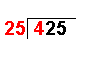4 ÷ 25 = 0 remainder 4 The first digit of the dividend (4) is divided by the divisor.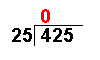The whole number result is placed at the top. Any remainders are ignored at this point.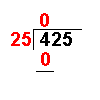25 × 0 = 0 The answer from the first operation is multiplied by the divisor. The result is placed under the number divided into.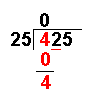4 − 0 = 4 Now we subtract the bottom number from the top number.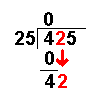Bring down the next digit of the dividend.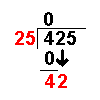42 ÷ 25 = 1 remainder 17 Divide this number by the divisor.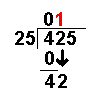The whole number result is placed at the top. Any remainders are ignored at this point.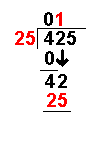25 × 1 = 25 The answer from the above operation is multiplied by the divisor. The result is placed under the last number divided into.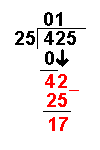42 − 25 = 17 Now we subtract the bottom number from the top number.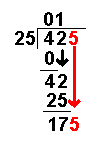Bring down the next digit of the dividend.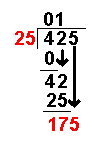175 ÷ 25 = 7 remainder 0 Divide this number by the divisor.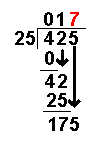The whole number result is placed at the top. Any remainders are ignored at this point.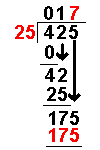25 × 7 = 175 The answer from the above operation is multiplied by the divisor. The result is placed under the number divided into.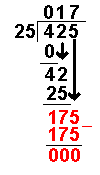175 − 175 = 0 Now we subtract the bottom number from the top number. There are no more digits to bring down. The answer must be 17

Subpages (2):